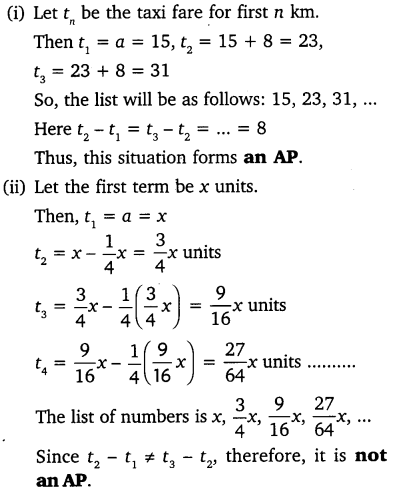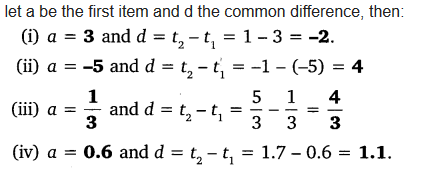# NCERT Solutions for Class 10 Maths Chapter 5 Arithmetic Progressions Ex 5.1

Get Free NCERT Solutions for Class 10 Maths Chapter 5 Ex 5.1 PDF. Arithmetic Progressions Class 10 Maths NCERT Solutions are extremely helpful while doing your homework. Exercise 5.1 Class 10 Maths NCERT Solutions were prepared by Experienced LearnCBSE.in Teachers. Detailed answers of all the questions in Chapter 5 Maths Class 10 Arithmetic Progressions Exercise 5.1 provided in NCERT TextBook.

Topics and Sub Topics in Class 10 Maths Chapter 5 Arithmetic Progressions:

 Section Name Topic Name 5 Arithmetic Progressions 5.1 Introduction 5.2 Arithmetic Progressions 5.3 Nth Term Of An AP 5.4 Sum Of First N Terms Of An AP 5.5 Summary

## NCERT Solutions for Class 10 Maths Chapter 5 Arithmetic Progressions Ex 5.1

NCERT Solutions for Class 10 Maths Chapter 5 Arithmetic Progressions Ex 5.1 are part of NCERT Solutions for Class 10 Maths. Here we have given NCERT Solutions for Class 10 Maths Chapter 5 Arithmetic Progressions Exercise 5.1

 Board CBSE Textbook NCERT Class Class 10 Subject Maths Chapter 5 Chapter Name Arithmetic Progressions Exercise Ex 5.1 Number of Questions Solved 4 Category NCERT Solutions

We also solved 106 questions from Chapter 9 – Arithmetic Progressions of RD Sharma Class 10 Maths Textbook.

Page No: 99

Question 1.
In which of the following situations, does the list of numbers involved make an arithmetic progression and why?
(i) The taxi fare after each km when the fare is ₹ 15 for the first km and ₹ 8 for each additional km.
(ii) The amount of air present in a cylinder when a vacuum pump removes$\frac { 1 }{ 4 }$of the air remaining in the cylinder at a time.
(iii) The cost of digging a well after every meter of digging, when it costs ₹ 150 for the first meter and rises by ₹ 50 for each subsequent meter.
(iv) The amount of money in the account every year, when ₹ 10000 is deposited at compound interest at 8% per annum.
Solution:Question 2.
Write first four terms of the AP, when the first term a and the common difference d are given as follows:
(i) a = 10, d = 10
(ii) a = -2, d = 0
(iii) a = 4, d = -3
(iv) a = -1, d =$\frac { 1 }{ 2 }$
(v) a = -1.25, d = -0.25
Solution:You can also download the free PDF of  Ex 5.1 Class 10 Arithmetic Progressions NCERT Solutions or save the solution images and take the print out to keep it handy for your exam preparation.

Question 3.
For the following APs, write the first term and the common difference:
(i) 3, 1, -1, -3, ……
(ii) -5, -1, 3, 7, ……
(iii)$\frac { 1 }{ 3 }$,$\frac { 5 }{ 3 }$,$\frac { 9 }{ 3 }$,$\frac { 13 }{ 3 }$, ……..
(iv) 0.6, 1.7, 2.8, 3.9, …….
Solution:Question 4.
Which of the following are APs ? If they form an AP, find the common difference d and write three more terms.
(i) 2, 4, 8, 16, …….
(ii) 2,$\frac { 5 }{ 2 }$, 3,$\frac { 7 }{ 2 }$, …….
(iii) -1.2, -3.2, -5.2, -7.2, ……
(iv) -10, -6, -2,2, …..
(v) 3, 3 +$\sqrt { 2 }$, 3 + 2$\sqrt { 2 }$, 3 + 3$\sqrt { 2 }$, …..
(vi) 0.2, 0.22, 0.222, 0.2222, ……
(vii) 0, -4, -8, -12, …..
(viii)$\frac { -1 }{ 2 }$,$\frac { -1 }{ 2 }$,$\frac { -1 }{ 2 }$,$\frac { -1 }{ 2 }$, …….
(ix) 1, 3, 9, 27, …….
(x) a, 2a, 3a, 4a, …….
(xi) a, a2, a3, a4, …….
(xii)$\sqrt { 2 }$,$\sqrt { 8 }$,$\sqrt { 18 }$,$\sqrt { 32 }$, …..
(xiii)$\sqrt { 3 }$,$\sqrt { 6 }$,$\sqrt { 9 }$,$\sqrt { 12 }$, …..
(xiv) 12, 32, 52, 72, ……
(xv) 12, 52, 72, 73, ……
Solution:error: Content is protected !!
+